oomph::GeneralisedHookean Class Reference

////////////////////////////////////////////////////////////////////// ////////////////////////////////////////////////////////////////////// More...

#include <constitutive_laws.h>Inheritance diagram for oomph::GeneralisedHookean:

## Public Member Functions

GeneralisedHookean (double *nu_pt, double *e_pt)
The constructor takes the pointers to values of material parameters: Poisson's ratio and Young's modulus. More...

GeneralisedHookean (double *nu_pt)
The constructor takes the pointers to value of Poisson's ratio . Young's modulus is set to E=1.0, implying that all stresses have been non-dimensionalised on on it. More...

virtual ~GeneralisedHookean ()
Virtual destructor. More...

void calculate_second_piola_kirchhoff_stress (const DenseMatrix< double > &g, const DenseMatrix< double > &G, DenseMatrix< double > &sigma)
Calculate the contravariant 2nd Piola Kirchhoff stress tensor. Arguments are the covariant undeformed and deformed metric tensor and the matrix in which to return the stress tensor. More...

void calculate_second_piola_kirchhoff_stress (const DenseMatrix< double > &g, const DenseMatrix< double > &G, DenseMatrix< double > &sigma_dev, DenseMatrix< double > &G_contra, double &Gdet)
Calculate the deviatoric part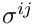of the contravariant 2nd Piola Kirchhoff stress tensor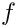. Also return the contravariant deformed metric tensor and the determinant of the deformed metric tensor. This form is appropriate for truly-incompressible materials for which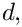where the "pressure"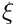is determined by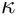. More...

void calculate_second_piola_kirchhoff_stress (const DenseMatrix< double > &g, const DenseMatrix< double > &G, DenseMatrix< double > &sigma_dev, DenseMatrix< double > &Gcontra, double &gen_dil, double &inv_kappa)
Calculate the deviatoric part of the contravariant 2nd Piola Kirchoff stress tensor. Also return the contravariant deformed metric tensor, the generalised dilatation,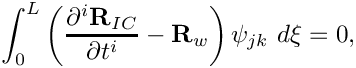and the inverse of the bulk modulus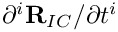. This form is appropriate for near-incompressible materials for which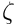where the "pressure"is determined from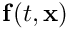. More...

bool requires_incompressibility_constraint ()
Pure virtual function in which the writer must declare if the constitutive equation requires an incompressible formulation in which the volume constraint is enforced explicitly. Used as a sanity check in PARANOID mode. False. More...Public Member Functions inherited from oomph::ConstitutiveLaw
ConstitutiveLaw ()
Empty constructor. More...

virtual ~ConstitutiveLaw ()
Empty virtual destructor. More...

virtual void calculate_d_second_piola_kirchhoff_stress_dG (const DenseMatrix< double > &g, const DenseMatrix< double > &G, const DenseMatrix< double > &sigma, RankFourTensor< double > &d_sigma_dG, const bool &symmetrize_tensor=true)
Calculate the derivatives of the contravariant 2nd Piola Kirchhoff stress tensor with respect to the deformed metric tensor. Arguments are the covariant undeformed and deformed metric tensor, the current value of the stress tensor and the rank four tensor in which to return the derivatives of the stress tensor The default implementation uses finite differences, but can be overloaded for constitutive laws in which an analytic formulation is possible. If the boolean flag symmetrize_tensor is false, only the "upper triangular" entries of the tensor will be filled in. This is a useful efficiency when using the derivatives in Jacobian calculations. More...

virtual void calculate_d_second_piola_kirchhoff_stress_dG (const DenseMatrix< double > &g, const DenseMatrix< double > &G, const DenseMatrix< double > &sigma, const double &detG, const double &interpolated_solid_p, RankFourTensor< double > &d_sigma_dG, DenseMatrix< double > &d_detG_dG, const bool &symmetrize_tensor=true)
Calculate the derivatives of the contravariant 2nd Piola Kirchhoff stress tensor. with respect to the deformed metric tensor. Also return the derivatives of the determinant of the deformed metric tensor with respect to the deformed metric tensor. This form is appropriate for truly-incompressible materials. The default implementation uses finite differences for the derivatives that depend on the constitutive law, but not for the derivatives of the determinant, which are generic. / If the boolean flag symmetrize_tensor is false, only the "upper triangular" entries of the tensor will be filled in. This is a useful efficiency when using the derivatives in Jacobian calculations. More...

virtual void calculate_d_second_piola_kirchhoff_stress_dG (const DenseMatrix< double > &g, const DenseMatrix< double > &G, const DenseMatrix< double > &sigma, const double &gen_dil, const double &inv_kappa, const double &interpolated_solid_p, RankFourTensor< double > &d_sigma_dG, DenseMatrix< double > &d_gen_dil_dG, const bool &symmetrize_tensor=true)
Calculate the derivatives of the contravariant 2nd Piola Kirchoff stress tensor with respect to the deformed metric tensor. Also return the derivatives of the generalised dilatation,with respect to the deformed metric tensor. This form is appropriate for near-incompressible materials. The default implementation uses finite differences. If the boolean flag symmetrize_tensor is false, only the "upper triangular" entries of the tensor will be filled in. This is a useful efficiency when using the derivatives in Jacobian calculations. More...

## Private Attributes

double * Nu_pt
Poisson ratio. More...

double * E_pt
Young's modulus. More...

bool Must_delete_e
Boolean flag to indicate if storage for elastic modulus must be deleted in destructor. More...Protected Member Functions inherited from oomph::ConstitutiveLaw
bool is_matrix_square (const DenseMatrix< double > &M)
Test whether a matrix is square. More...

bool are_matrices_of_equal_dimensions (const DenseMatrix< double > &M1, const DenseMatrix< double > &M2)
Test whether two matrices are of equal dimensions. More...

void error_checking_in_input (const DenseMatrix< double > &g, const DenseMatrix< double > &G, DenseMatrix< double > &sigma)
Check for errors in the input, i.e. check that the dimensions of the arrays are all consistent. More...

double calculate_contravariant (const DenseMatrix< double > &Gcov, DenseMatrix< double > &Gcontra)
Calculate a contravariant tensor from a covariant tensor, and return the determinant of the covariant tensor. More...

void calculate_d_contravariant_dG (const DenseMatrix< double > &Gcov, RankFourTensor< double > &dGcontra_dG, DenseMatrix< double > &d_detG_dG)
Calculate the derivatives of the contravariant tensor and the derivatives of the determinant of the covariant tensor with respect to the components of the covariant tensor. More...

## Detailed Description

////////////////////////////////////////////////////////////////////// //////////////////////////////////////////////////////////////////////

Class for a "non-rational" extension of classical linear elasticity to large displacements: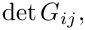where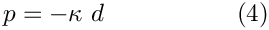For small strainsthis approaches the version appropriate for linear elasticity, obtained by replacing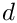with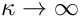.

We provide three versions of calculate_second_piola_kirchhoff_stress():

1. If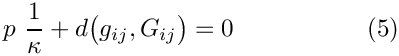(and not close to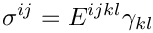), the constitutive law can be used directly in the above form, using the deformed and undeformed metric tensors as input.
2. If the material is incompressible (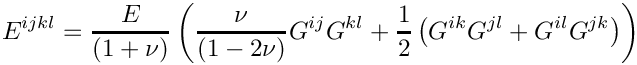), the first term in the above expression for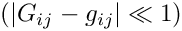is singular. We re-write the constitutive equation for this case as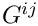where the pressureneeds to be determined independently via the incompressibility constraint. In this case, the stress returned by calculate_second_piola_kirchhoff_stress() contains only the deviatoric part of the 2nd Piola Kirchhoff stress,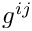The function also returns the contravariant metric tensor(since it is needed to form the complete stress tensor), and the determinant of the deformed covariant metric tensor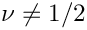(since it is needed in the equation that enforces the incompressibility).

3. If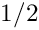, the original form of the constitutive equation could be used, but the resulting equations tend to be ill-conditioned since they contain the product of the large "bulk modulus"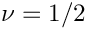and the small "generalised dilatation"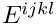[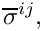represents the actual dilatation in the small strain limit; for large deformations it doesn't have any sensible interpretation (or does it?). It is simply the term that needs to go to zero as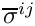.] In this case, the stress returned by calculate_second_piola_kirchhoff_stress() contains only the deviatoric part of the 2nd Piola Kirchhoff stress,The function also returns the contravariant metric tensor(since it is needed to form the complete stress tensor), the inverse of the bulk modulus, and the generalised dilatation (since they are needed in the equation that determines the pressure).

Definition at line 698 of file constitutive_laws.h.

## ◆ GeneralisedHookean() [1/2]

 oomph::GeneralisedHookean::GeneralisedHookean ( double * nu_pt, double * e_pt )
inline

The constructor takes the pointers to values of material parameters: Poisson's ratio and Young's modulus.

Definition at line 703 of file constitutive_laws.h.

## ◆ GeneralisedHookean() [2/2]

 oomph::GeneralisedHookean::GeneralisedHookean ( double * nu_pt )
inline

The constructor takes the pointers to value of Poisson's ratio . Young's modulus is set to E=1.0, implying that all stresses have been non-dimensionalised on on it.

Definition at line 712 of file constitutive_laws.h.

## ◆ ~GeneralisedHookean()

 virtual oomph::GeneralisedHookean::~GeneralisedHookean ( )
inlinevirtual

Virtual destructor.

Definition at line 722 of file constitutive_laws.h.

References E_pt, and Must_delete_e.

## ◆ calculate_second_piola_kirchhoff_stress() [1/3]

 void oomph::GeneralisedHookean::calculate_second_piola_kirchhoff_stress ( const DenseMatrix< double > & g, const DenseMatrix< double > & G, DenseMatrix< double > & sigma )
virtual

Calculate the contravariant 2nd Piola Kirchhoff stress tensor. Arguments are the covariant undeformed and deformed metric tensor and the matrix in which to return the stress tensor.

////////////////////////////////////////////////////////////////// //////////////////////////////////////////////////////////////////

Calculate the contravariant 2nd Piola Kirchhoff stress tensor. Arguments are the covariant undeformed (stress-free) and deformed metric tensors, g and G, and the matrix in which to return the stress tensor.

Implements oomph::ConstitutiveLaw.

Definition at line 682 of file constitutive_laws.cc.

Referenced by calculate_second_piola_kirchhoff_stress().

## ◆ calculate_second_piola_kirchhoff_stress() [2/3]

 void oomph::GeneralisedHookean::calculate_second_piola_kirchhoff_stress ( const DenseMatrix< double > & g, const DenseMatrix< double > & G, DenseMatrix< double > & sigma_dev, DenseMatrix< double > & G_contra, double & Gdet )
virtual

Calculate the deviatoric partof the contravariant 2nd Piola Kirchhoff stress tensor. Also return the contravariant deformed metric tensor and the determinant of the deformed metric tensor. This form is appropriate for truly-incompressible materials for whichwhere the "pressure"is determined by.

Reimplemented from oomph::ConstitutiveLaw.

Definition at line 811 of file constitutive_laws.cc.

## ◆ calculate_second_piola_kirchhoff_stress() [3/3]

 void oomph::GeneralisedHookean::calculate_second_piola_kirchhoff_stress ( const DenseMatrix< double > & g, const DenseMatrix< double > & G, DenseMatrix< double > & sigma_dev, DenseMatrix< double > & Gcontra, double & gen_dil, double & inv_kappa )
virtual

Calculate the deviatoric part of the contravariant 2nd Piola Kirchoff stress tensor. Also return the contravariant deformed metric tensor, the generalised dilatation,and the inverse of the bulk modulus. This form is appropriate for near-incompressible materials for whichwhere the "pressure"is determined from.

Reimplemented from oomph::ConstitutiveLaw.

Definition at line 765 of file constitutive_laws.cc.

## ◆ requires_incompressibility_constraint()

 bool oomph::GeneralisedHookean::requires_incompressibility_constraint ( )
inlinevirtual

Pure virtual function in which the writer must declare if the constitutive equation requires an incompressible formulation in which the volume constraint is enforced explicitly. Used as a sanity check in PARANOID mode. False.

Implements oomph::ConstitutiveLaw.

Definition at line 773 of file constitutive_laws.h.

## ◆ E_pt

 double* oomph::GeneralisedHookean::E_pt
private

Young's modulus.

Definition at line 783 of file constitutive_laws.h.

Referenced by calculate_second_piola_kirchhoff_stress(), and ~GeneralisedHookean().

## ◆ Must_delete_e

 bool oomph::GeneralisedHookean::Must_delete_e
private

Boolean flag to indicate if storage for elastic modulus must be deleted in destructor.

Definition at line 787 of file constitutive_laws.h.

Referenced by ~GeneralisedHookean().

## ◆ Nu_pt

 double* oomph::GeneralisedHookean::Nu_pt
private

Poisson ratio.

Definition at line 780 of file constitutive_laws.h.

Referenced by calculate_second_piola_kirchhoff_stress().

The documentation for this class was generated from the following files: